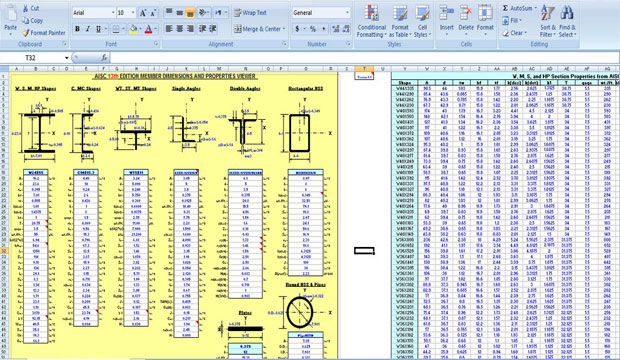Download Concrete Estimating SpreadsheetsTo determine what kind of concrete and how much concrete is necessary for the construction work you need some important spreadsheets to perform the task. And you also need a concrete calculator to do the calculating tasks.
Here we provide an excel sheet that contains:
• Concrete Continuous Footing Quantity Take-off Worksheet
• Concrete Spread Footing Quantity Take-off Worksheet
• Concrete Slab Quantity Take-off Worksheet
• Concrete Wall Quantity Take-off Worksheet
• Concrete Beam Quantity Take-off Worksheet
• Concrete Column Quantity Take-off Worksheet
• Concrete Calculator